# Combinatorial Optimization

## Theory and Algorithms

#### Algorithms and Combinatorics 21 Springer-Verlag, Berlin Heidelberg New York Tokyo Paris Milano

• First Edition 2000
• Second Edition 2002
• Japanese Edition 2005
• Third Edition 2006
• Fourth Edition 2008
• German Edition 2008
• Second Japanese Edition 2009
• French Edition 2010
• Italian Edition 2011
• Fifth Edition 2012
• Second German Edition 2012
• Chinese Edition 2014 (Science Press China)
• Russian Edition 2015 (Moscow Center for Continuous Mathematical Education)
• Second French Edition 2018
• Sixth Edition 2018
• Third German Edition 2018
• Third Japanese Edition 2022 (forthcoming)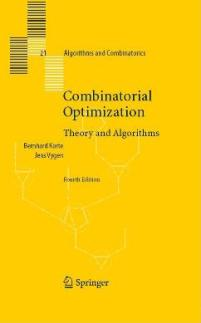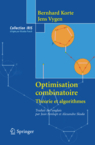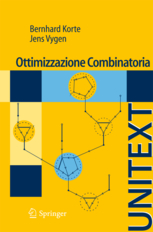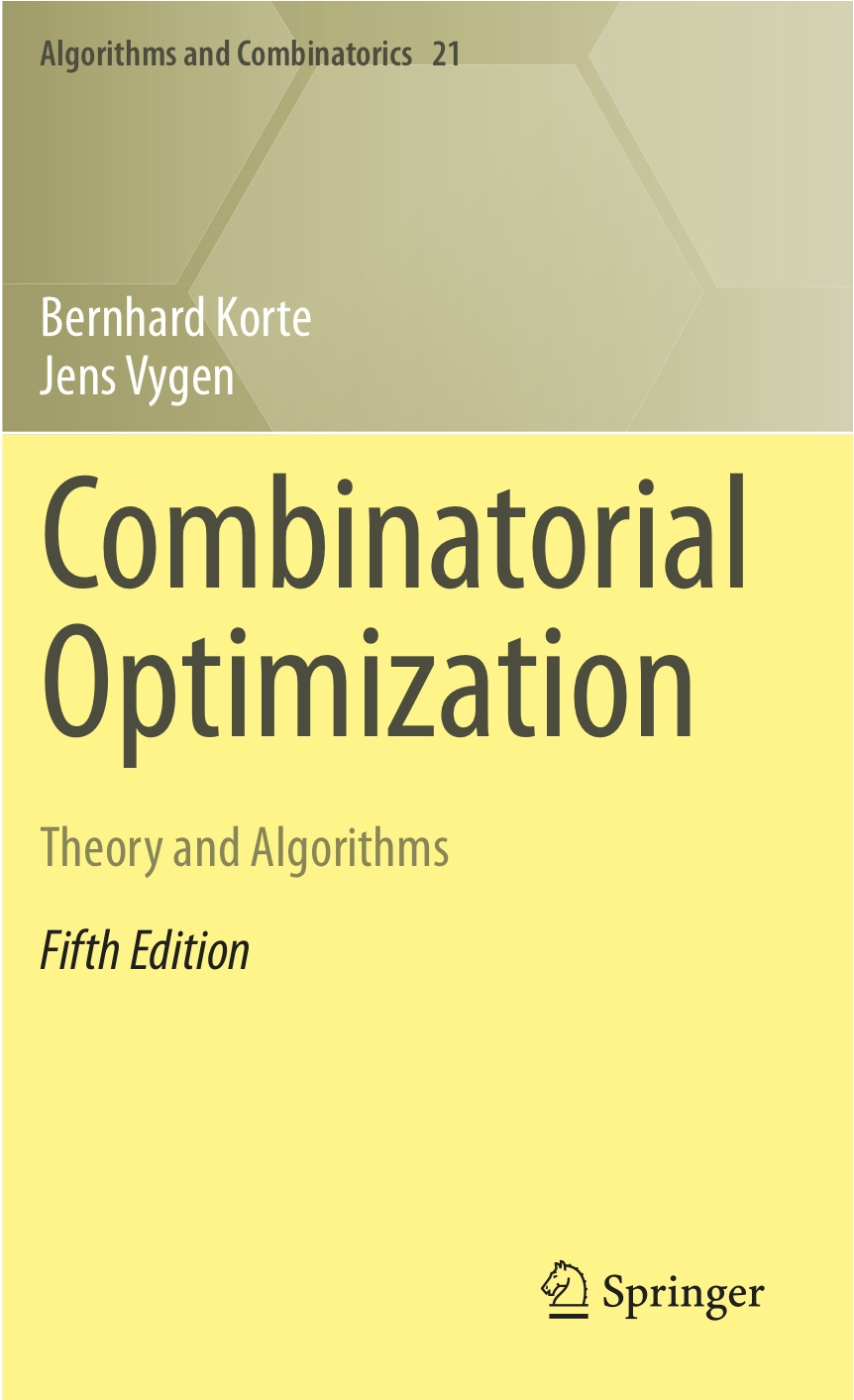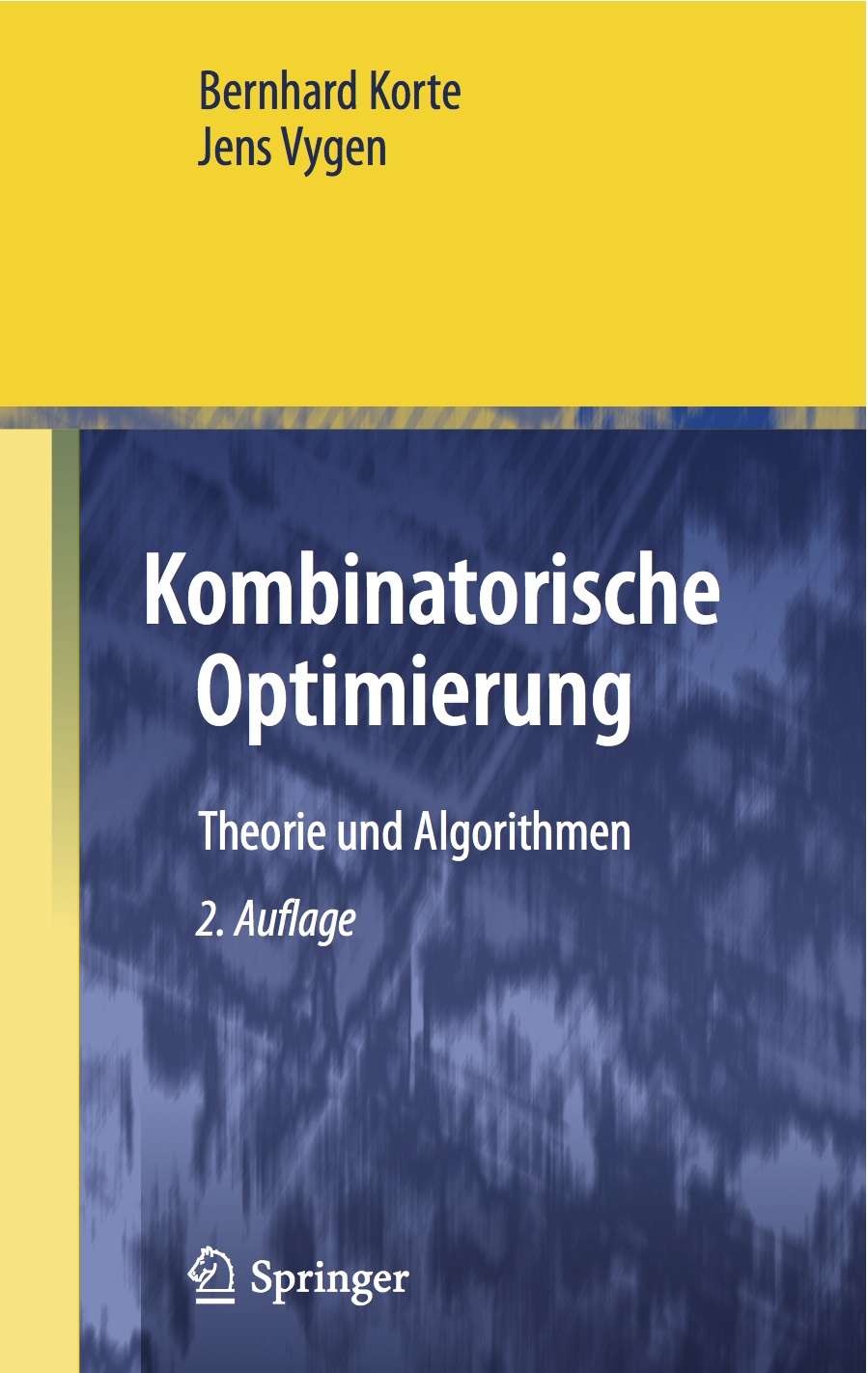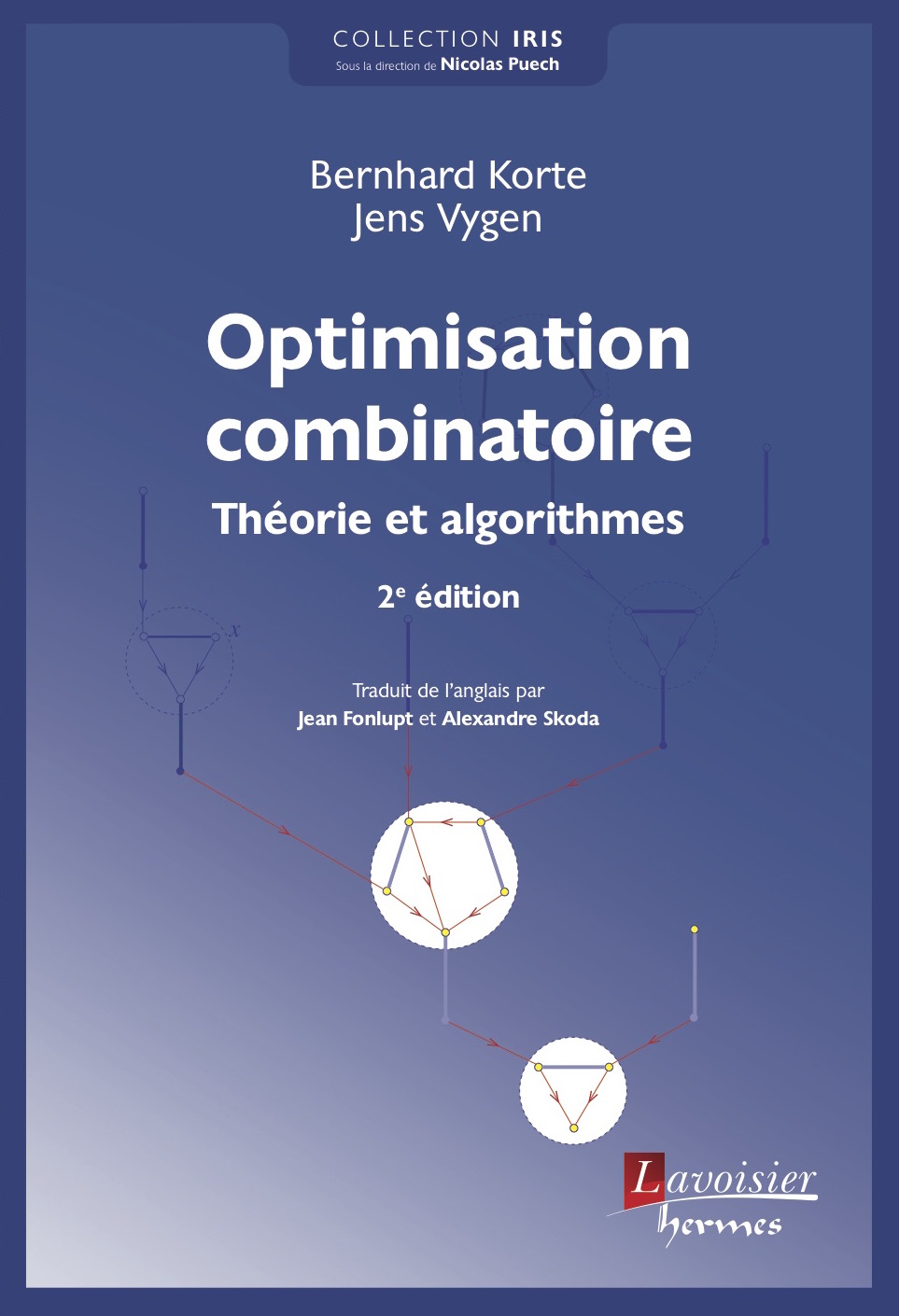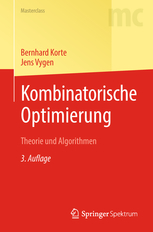# List of Updates and Errors of the Sixth Edition

All entries in the following list refer to the sixth edition. (For an outdated list for the 5th edition, see here.) Any additional comments are welcome.

Page Line Comment
38 24 Replace 1958 by 1758.
58 14 Replace "min" by "max".
153 13-16 The inequality system in Exercise 20 is not correct. A correct version is: $x_e\ge 0$ ($e\in E(G)$), $z_{r,u,v}\ge 0$ ($\{u,v\}\in E(G), r\in V(G)$), $\sum_{e\in E(G)}x_e=n-1$, $x_e=z_{r,u,v}+z_{r,v,u}$ ($e=\{u,v\}\in E(G)$, $r\in V(G)$), and $\sum_{\{u,v\}\in\delta(v)} z_{r,u,v}=1$ ($v\in V(G)$, $r\in V(G)\setminus \{v\}$).
204 24 One must require $c \ge c'$ in Exercise 6.
303 25-27 The full version of the paper by Gabow  appeared in ACM Transactions on Algorithms 14 (2018), Article 39.
324 1-3 The full version of the paper by Gabow  appeared in ACM Transactions on Algorithms 14 (2018), Article 39.
354 31 Rep[lace "r(X)=" by "r'(X)=".
378 10 The right-hand side of this inequality should be $\max \{ \sum_{t=(p,A,B)\in T_{i-1}} p x_t \Delta_B, \sum_{t=(p,A,B)\in T_{i-1}} p (1-x_t) \Delta_A \}$. This is sufficient because either $\Exp[f(O_{i-1})-f(O_i)] \le x_t \Delta_B$ for all $(p,A,B)\in T_{i-1}$ or $\Exp[f(O_{i-1})-f(O_i)] \le (1-x_t) \Delta_A$ for all $(p,A,B)\in T_{i-1}$; see the proof of (14.4).
379 32 In Exercise 10, one must require $f(\emptyset)=0$.
379 38 In Exercise 11,  f  should be integer-valued.
381 30 Replace "$f$ and any $g_C$" by "$g$ and any $f_C$".
382 26-28 The paper by Buchbinder and Feldman  appeared in ACM Transactions on Algorithms 14 (2018), Article 14.
434 3 A factor 1/2 is missing on the left-hand side of the inequality.
460 21-22 The half-sentence "which is better..." makes no sense and should be deleted.
480 7 Replace (U,V,W,T) by (P,Q,R,T).
533 29 This line should read: "Set $E(G):=\delta_{G'}(X)$ and $E(H):=E(G')\setminus\delta_{G'}(X)$."
552 13 Replace G+f by G[T]+f.
557 21 We can choose $Y^*_k$ to be an optimum Steiner tree here.
558 13 The second sum should of course go over $C\in\delta^+_{\mathcal{C}^k}(U)$.
573/574 19/25 Lemma 20.38 and Theorem 20.39 also hold when f can have negative values (which can happen when we apply the Theorem).
584 33 We set $x'_e:=\frac{1}{1-\alpha}x_e$.
628 28-29 The paper by Svensson, Tarnawski and Végh  appeared in the Journal of the ACM 67 (2020), Article 37.
628 30-32 The paper by Traub and Vygen  appeared in the Journal of the ACM 66 (2019), Article 14.
630 29-30 "greater than 1" should be replaced by "greater than 2".

Last change: Feburary 3, 2023. Thanks to Jannis Blauth, Lukas Bonfert, Ulrich Brenner, Marc Goerigk, Stephan Held, Felix Horchler, Solomon Lo, Stefan Rabenstein, Rabe von Randow, Rudolf Scheifele, Jannik Silvanus, Vera Traub, Shi-Chun Tsai, Dingwen Yuan, and all people reporting errors in the future!